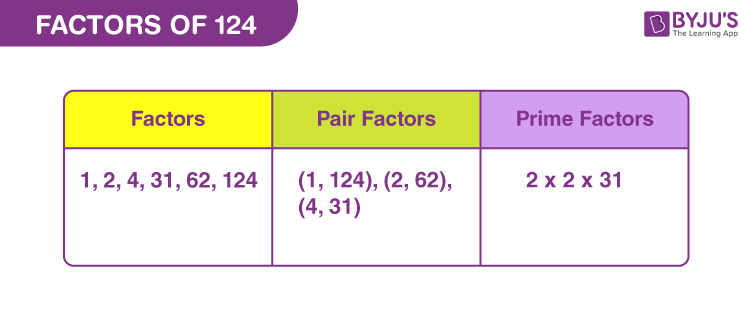# Factors of 124

In Mathematics, factors of 124 are the natural numbers that divide the original number, without leaving a remainder. The factors are also called the divisors of the number. Since, 124 is an even composite number, thus the factors are more than two for it.

If the product of two natural numbers results in 124, then they are the required factors. Now to determine these factors we can use either the division method or multiplication method. The prime factors of 124 are determined using the prime factorisation method.## How to Find the Factors of 124?

A factor divides the original number, uniformly. Therefore, we can find the factors of 124 by dividing it by other natural numbers.

• 124 ÷ 1 = 124
• 124 ÷ 2 = 62
• 124 ÷ 4 = 31
• 124 ÷ 31 = 4
• 124 ÷ 62 = 2
• 124 ÷ 124 = 1

Therefore, the factors of 124: 1, 2, 4, 31, 62 and 124.

## Pair Factors of 124

The factors of 124, when multiplied in a particular pair to get the original number, are called the pair factors or factors in pair.

• 1 × 124 = 124
• 2 × 62 = 124
• 4 × 31 = 124

Therefore, the pair factors of 124 are (1, 124), (2, 62) and (4, 31).

If we multiply the pair factors of 124 with negative sign, then also we get the original number.

• -1 × -124 = 124
• -2 × -62 = 124
• -4 × -31 = 124

Therefore, the negative pair factors are (-1, -124), (-2, -62) and (-4, -31).

## Prime Factorisation of 124

The prime factors of 124 can be evaluated by prime factorising the original number. Here we factorise 124 by dividing it by prime numbers till the final result is 1.

 124 ÷ 2 = 62 62 ÷ 2 = 31 31 ÷ 31 = 1

Therefore, the prime factor of 124 is 2 and 31.

 Prime factorisation of 124 = 2 x 2 x 31 Exponential form = 22 × 311

## Solved Examples

Q.1: What should be multiplied by 31 to get 124?

Solution: Suppose, x is the number to be multiplied by 31 to get 124.

So, x × 21 = 124

x = 124/31

x = 4

Therefore, 4 should be multiplied by 31 to get 124.

Q.2: What is the sum of factors of 124?

Solution: The factors of number 124 are 1, 2, 4, 31, 62, 124.

Sum of all the factors = 1 + 2 + 4 + 31 + 62 + 124

Sum = 224

Therefore, 224 is the required sum.

Q.3: What is the GCF of 124 and 130?

Solution: The factors of 120 and 124, respectively, are:

124 → 1, 2, 4, 31, 62, 124

130 → 1, 2, 5, 10, 13, 26, 65, 130

Therefore, the greatest common factor of 124 and 130 is:

GCF (124, 130) = 2

Q.4: What are the common factors of 124 and 144?

Solution: The factors of the respective numbers are:

124 → 1, 2, 4, 31, 62, 124

144 → 1, 2, 3, 4, 6, 8, 9, 12, 16, 18, 24, 36, 48, 72, 144

The common factors are 1, 2 and 4.

### Practice Questions

1. What are the even divisors of 124?
2. In how many ways can 124 be divided evenly?
3. Find the common factors of 124 and 132.
4. What is the GCF of 100 and 124?

## Frequently Asked Questions on Factors of 124

### What are the factors of 124?

The factors of 124 are 1, 2, 4, 31, 62 and 124. Hence, there are a total of six factors of 124.

### What are the multiples of 124?

The first ten multiples of 124 are 124, 248, 372, 496, 620, 744, 868, 992, 1116 and 1240.

### Is 124 a prime number or composite number?

124 is a composite number not a prime number. It has factors more than two.

### What is the HCF of 124 and 24?

The highest common factor of 124 and 24 is 4.

### What is the GCF of 124 and 300?

The GCF (Greatest common factor) of 124 and 300 is 4.

### Is 124 a perfect square?

124 is not a perfect square.